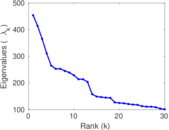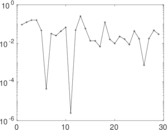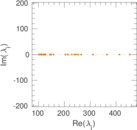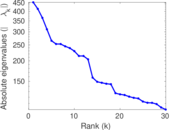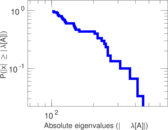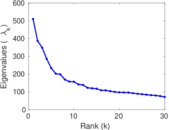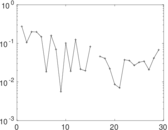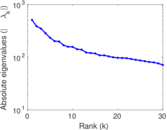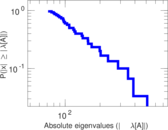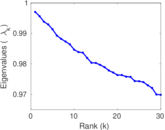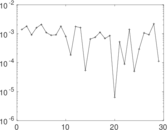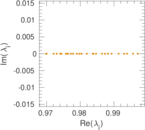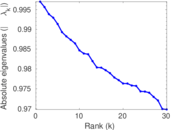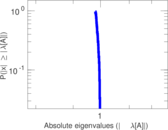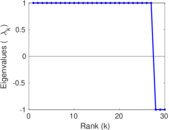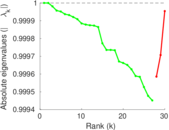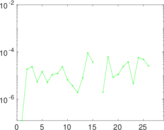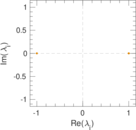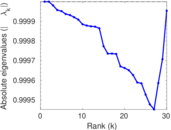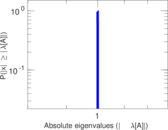This network consists of the wikilinks of the Wikipedia in the Chinese (Min Nan) language (zh-min-nan). Nodes are Wikipedia articles, and directed edges are wikilinks, i.e., hyperlinks within one wiki. In the wiki source, these are indicated with [[double brackets]]. Only pages in the article namespace are included.

 Code `Wzh-min-nan` Internal name `wikipedia_link_zh_min_nan` Name Wikipedia links (zh-min-nan) Data source http://dumps.wikimedia.org/ AvailabilityDataset is available for download Consistency checkDataset passed all tests Category Hyperlink network Node meaning Article Edge meaning Wikilink Network format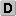Unipartite, directed Edge typeUnweighted, no multiple edges ReciprocalContains reciprocal edges Directed cyclesContains directed cycles Loops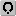Contains loops

## Statistics

 Size n = 429,317 Volume m = 4,421,679 Loop count l = 145 Wedge count s = 17,692,846,133 Claw count z = 377,632,019,424,593 Cross count x = 8,195,151,838,068,425,728 Triangle count t = 90,790,035 Square count q = 41,994,257,123 Maximum degree dmax = 111,978 Maximum outdegree d+max = 814 Maximum indegree d−max = 111,949 Average degree d = 20.598 7 Fill p = 2.399 00 × 10−5 Size of LCC N = 429,150 Diameter δ = 11 50-Percentile effective diameter δ0.5 = 3.363 97 90-Percentile effective diameter δ0.9 = 4.748 60 Median distance δM = 4 Mean distance δm = 3.857 70 Gini coefficient G = 0.834 756 Balanced inequality ratio P = 0.164 151 Outdegree balanced inequality ratio P+ = 0.223 003 Indegree balanced inequality ratio P− = 0.140 221 Power law exponent γ = 1.784 17 Tail power law exponent γt = 1.951 00 Degree assortativity ρ = −0.095 837 1 Degree assortativity p-value pρ = 0.000 00 In/outdegree correlation ρ± = +0.801 425 Clustering coefficient c = 0.015 394 4 Directed clustering coefficient c± = 0.853 859 Spectral norm α = 915.597 Operator 2-norm ν = 510.118 Cyclic eigenvalue π = 454.000 Algebraic connectivity a = 0.017 484 6 Spectral separation |λ1[A] / λ2[A]| = 1.102 42 Reciprocity y = 0.576 491 Non-bipartivity bA = 0.445 746 Normalized non-bipartivity bN = 0.001 492 00 Spectral bipartite frustration bK = 0.000 247 197

## Plots

### Degree distribution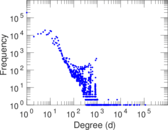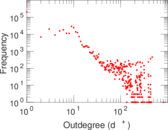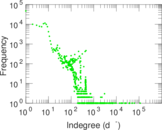### Cumulative degree distribution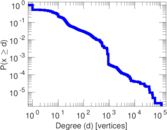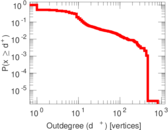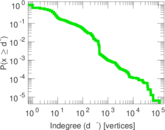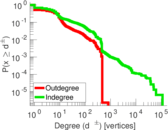### Lorenz curve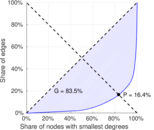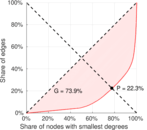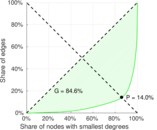### Degree assortativity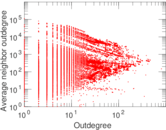### Zipf plot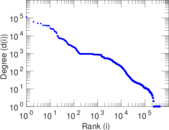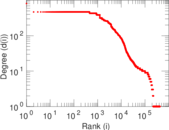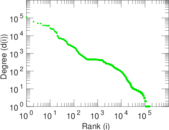### Hop distribution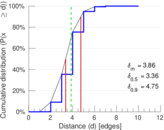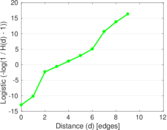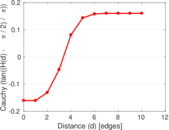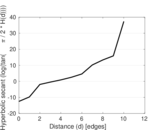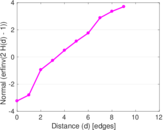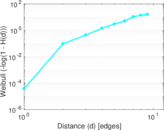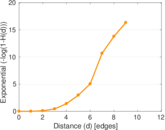### Delaunay graph drawing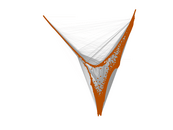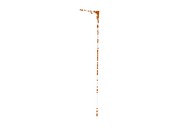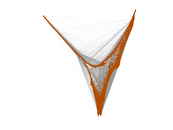### In/outdegree scatter plot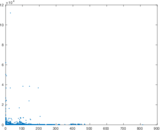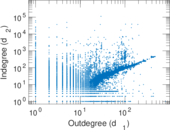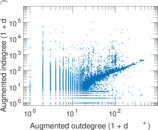### SynGraphy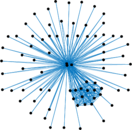### Matrix decompositions plots